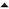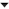## H-bridge for controlling phone bell

Because my post about the old school phone bell was getting a bit to big, i decided to make this a separate post.

A H-brige is generally used to power DC motors and change direction. Its build with 4 FETs and some additional components. My knowledge of analog electronics was a bit rusty but the guys on the forum here where a great help. Below you can see a picture of just the H-bridge. This is just one solution, and I’m sure there are better/cheaper ways to design these, but I focused on using the best available parts and making it strong.

The way it works is simple. By supplying 5V to input A, the left P-FET turns on. By also powering input D, the right N-FET also turns on. There current can now flow trough both FET’s and trough the bell coil swinging the hammer left. If 5V is applied on input C and B, the right N-FET and left P-FET are powered. The current runs through both FETs and the bell coil, but now in the other direction swinging the hammer right. It is very important that A and B or C and D are never high at the same time. If this happens the power runs through both FET’s without passing the coil. This is basically shorting them. The MT3608 module will probably not supply enough current to blow them up, but other power supplies will.

You need both N-FETs and P-FETs for this schematic to work. N-FET’s will only turn on if Ugs (voltage between gate and source) is above its threshold. For the IRF-3205 FET’s I used this is approx. 4,5V. This means that when the we put 5V on the gate, while the source is connected to the ground, the N-FET will “turn on”. This is easy right? The problem is that this will not be so easy for the FETs that we need to connect to the 28V line. If the 28V is connected to the source, and we need a Ugs of 5V, we need to supply 28 + 5 = 32V. We do not have this voltage available, and the Arduino can only supply 5V. A solution can be found in a P-FET. These wil turn on when a negative Ugs voltage threshold is reached. In the case of the IRF9520 I used this is -4,5V. To turn on the gate in our H-bridge, we need to supply it with 28 – 5 = 23V (or less) To turn off the gate we need to make the Ugs close to 0V. This means the gate voltage needs to as close as the voltage on the source. In our case 28V.

This still makes it impossible to control the P-FET directly from the output of the Arduino, but now we can control it with the 28V we already have available. A simple NPN transistor with a resistor can be used to achieve this. I used a BC547. When we power the base of the transistor with 5V trough a 3300ohm resistor a current of (5-0,6)/3300 = 1,3mA runs through the base of the the transistor. The Hfe (amplification) of a BC547B is approx. 110, so the transistor will try to run 143mA trough the resistors connected to the collector. In my H-bridge the total value of the resistors is 32Kohm, so the voltage over these resistors will be 0,134 X 320000 = 4576V. The supplied voltage is only 28V so the transistor will basically turn on fully.

We have just 1 small challenge to solve. If we just uses a single resistor the gate of the connected P-FET will be pulled to ground. With a 12V power supply this would be fine and we would have a working H-bridge, but the Ugs of a FET has a maximum value. For the IRF9520 P-FET this value is -20V. If we supply 28V to its source, and 0V to its gate, the Ugs would be -28V and the FET would break down. That is why we have 2 resistors. This creates a voltage divider. A 2:1 ratio is fine for this purpose as the voltage over the smaller value resistor will be 1/3th of the supply voltage. In our case this is 9,3V. So now when we apply 5V to the NPN transistors base, the voltage on the gate of the P-FET will drop to 28-9,3 = 18.7V creating a Ugs of -9,3V. This is a totally save voltage for turn on the P-FET. To turn it of again, we remove the 5V from the base of the transistor. It will stop the current flow trough the resistors. The upper resistor will however allow the 28V to reach the gate so it turns off.11-'18 Old school phone as doorbell and domoitcs interface!02-'17 External Status LED's for Raspberry Pi (without GPIO)

#### Reacties

what happens when a woman takes a viagra pill canadian pharmacy viagra sildenafil eg 100 mg